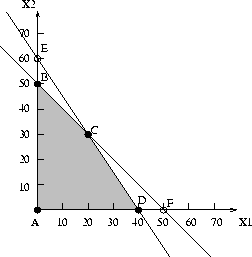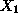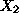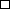Next: LP's in ``standard form'' Up: An algebraic characterization of Previous: An algebraic characterization of

#### Example:

We demonstrate the findings of the above discussion on the feasible region of the prototype example, which for convenience is reproduced in Figure 11.Figure 11: The feasible region of the prototype example LP

As we can see in the figure, each of the extreme points of this region corresponds to the binding of a pair of the LP constraints, i.e.,

• point A corresponds to the binding of the two sign restriction constraints,
• point B corresponds to the binding of the second technological constraint and the sign restriction of variable,
• point C corresponds to the binding of both technological constraints, and
• point D corresponds to the binding of the first technological constraint and the sign restriction of variable.

Notice, however, that points E and F, even though they are defined by the binding of the constraint pairs (tech. con. 1, sign res. of) and (tech. con. 2, sign res. of), respectively, are not extreme points of the feasible region just because they are infeasible (i.e., some other LP constraints are violated). Hence, having n linearly independent constraints binding at certain point is a necessary condition for it to be an extreme point of the feasible region, but not sufficient.Finally, it should be easy to see that for an n-var LP, n is the minimum number of constraints binding at an extreme point. If more than this minimum number of constraints are binding to an extreme point, the point (and the corresponding solution) are characterized as degenerate. Degeneracy can complicate the search for the optimal solution carried out by the Simplex method.

UAL Data
Fri Jun 20 15:03:05 CDT 1997